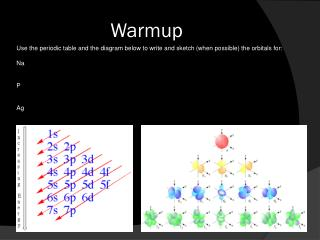Download PresentationWarmup# Warmup - PowerPoint PPT Presentation

Download Presentation##### Warmup

Download Policy: Content on the Website is provided to you AS IS for your information and personal use and may not be sold / licensed / shared on other websites without getting consent from its author. While downloading, if for some reason you are not able to download a presentation, the publisher may have deleted the file from their server.

- - - - - - - - - - - - - - - - - - - - - - - - - - - E N D - - - - - - - - - - - - - - - - - - - - - - - - - - -
##### Presentation Transcript

1. Warmup Use the periodic table and the diagram below to write and sketch (when possible) the orbitals for: Na P Ag

2. Warmup Solutions Na 1s2 2s2p6 3s1 P Ag

3. Warmup 2 Wave Equation An ocean wave has a velocity of 15 m/s and a wavelength of 30 m. What is the frequency in Hz? A light wave has a wavelength of 660 nm. What is its frequency? Planck’s Equation 3) h  Planck’s Constant (6.63 * 10-34 J*s) f  frequency (Hz) use answer from problem 2. What is the energy of this photon?

4. SEPTEMBER 20TH 2010 Electron Orbitals 2 • Bohr Model: Quantized Orbits • Rydberg Formula • Emission Spectra • Flame Test

5. Bohr Model: Quantized Orbits • Main Principles: • Atoms have discrete electron orbital patterns. • Higher orbits hold more potential energy. • A fall from a higher orbital to a lower one releases energy as light. • (Energy is conserved) Ultraviolet Infrared Infrared Infrared Visible http://www.physchem.co.za/OB12-mat/spectra.htm

6. Relevant Equations Potential Energy PE = F * d PE  Potential Energy (J) F  Force of attraction (N) d  Distance (m) Waves v = λ * f v  velocity (3*108 m/s) λ  wavelength (m) f  frequency (Hz) Quantization of Photons E = h * f E  Energy (J) h  Planck’s Constant (6.63 * 10-34 J*s) f  frequency (Hz) Rydberg Formula 1/ λ = R Z2 (n1-2 – n2-2)λ  wavelength (m) R  Rydberg’s Constant (1.1*10-7 m-1) Z  Atomic number n  electron orbital number

7. Flame Test Goals: 1) Measure the emission spectrum of a particular element. 2) Calculate the frequency and energy of the photons emitted. 3) Compare the measured wavelength to the Rydberg Formula prediction, and identify the line. Assumptions: 1) All visible lines are in the Balmer series (n=2). (Probably true) 2) Each element is ionized down to a single valence electron. (?!) Materials: Metal salts or nitrates Wire or heat-resistant ladles Propane torches Diffraction grating spectroscopes Backup: Spectroscopy tubes Resources: http://webmineral.com/help/FlameTest.shtml

8. Data Table Sample